# Building Autoregressive Models (Advanced Signal Processing Toolkit)

LabVIEW 2014 Advanced Signal Processing Toolkit Help

Edition Date: June 2014

Part Number: 372656C-01

»View Product InfoDownload Help (Windows Only)

Autoregressive (AR) models of a time series enable you to predict the current value xt of a time series, based on the past values xt–1, xt–2, ..., xt–n, plus a prediction error. The parameter n determines the number of past values you use to predict the current value. The following equation defines an AR model with an order of n:

xt + a1xt–1 + a2xt–2+ ... + anxt–n = et

where [1, a1, a2, ..., an] are the AR coefficients and et is the prediction error. Ideally, the residual prediction error is white noise with a mean value of zero.

You can rewrite the previous equation more concisely as follows:

A(q)xt = et

where A(q) is the AR operator, which is defined as follows:

A(q) = 1 + a1q–1 + a2q–2 + ... + anq–n

The term q–k is the backward shift operator, which is defined as follows:

q–kxt = xt–k

From a dynamic-system point of view, a time series is the response of a linear system with a white noise as the stimulus. An AR model or other models of the response signal describe the linear system. The prediction error of the model is the white noise et. The following figure shows a diagram that uses the AR model to describe a linear system.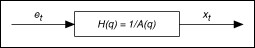H(q) represents the discrete-time transfer function of a physical system that generates the time series xt. Because H(q) is an AR model, it has only poles and no zeros. The roots of the polynomial A(q) are the poles of H(q). Therefore, after you estimate the AR model of a time series, you can use the resulting AR coefficients to estimate the dynamic characteristics of the system that generates the time series.Note  If a time series is generated by a linear system with a stimulus other than white noise, the characteristics of the stimulus become part of the response time series. After you build an AR model for the response time series, the AR model reflects the characteristics of both the linear system and the non-white stimulus.

Because many real-world linear systems can be modeled accurately with AR models, AR models are a good first choice for parametric modeling. The computation of AR models also is particularly efficient because in contrast with moving average (MA) and autoregressive-moving average (ARMA) models, you only need to compute linear-regression equations. Furthermore, the resulting model is unique and stable. AR models are numerically preferable to ARMA models, especially when the model order is high. However, AR models may not accurately model linear systems that do not have an AR response, or in cases where the measured time series is contaminated with noise or distortion. If an AR model is not appropriate, a high model order may be required to whiten the residual prediction error et. But if you use a high model order to force an AR model to fit a particular time series for which an AR model is not appropriate, you may get spurious spectral components in the resulting response.

For a multivariate time series with m variables, you can use an (m × 1)–length vector Xt to represent a multivariate time series, where XtT = (x1t, ..., xmt). To describe the interrelationship between these variables, you can extend Equation 5-1 to be a VAR model as follows:

Xt + A1Xt–1 + A2Xt–2 + ... + AnXt–n = Et

where n is the model order, I, A1, A2, ..., An are square matrices of the VAR coefficients, I is the identity matrix, the dimension of each matrix is m × m, and Et is the prediction-error vector, where EtT = (e1t, e2t, ..., emt). Each variable in Et ideally is white noise with a mean value of zero. If the model fit is good, these variables are not correlated with each other.

You can rewrite the previous equation concisely as follows:

A(q)Xt = Et

where A(q) is the AR operator, which is defined as follows:

A(q) = 1 + A1q–1 + A2q–2 + ... + Anq–n

The resulting VAR model of a multivariate time series is the coefficients matrix. However, you cannot obtain the dynamic characteristics of a multi-output system directly from the coefficients matrix of the VAR model. You must convert the model coefficients matrix into a state transition matrix in a stochastic state-space model. By computing the eigenvalues of a state transition matrix, you can obtain the poles of the system that generates the corresponding multivariate time series. You then can obtain the dynamic characteristics of the system from the poles.

Use the TSA AR Modeling VI or the Time Series Modeling Express VI to build AR and VAR models for univariate or multivariate time series.

## Selecting an Appropriate AR Order

The first step of estimating a model is to select an appropriate model. For a given model, selecting the model order is typically a trial-and-error process. Besides using background knowledge about the physical system that generates the time series, you also need to use other information, such as the information acquired from various statistical analysis methods, to justify the selected model order.

One tool for determining the model order is the partial auto-correlation function of the time series. The partial auto-correlation function is a function of lag. The partial auto-correlation value becomes very small when the lag equals a suitable AR order. The following figure shows an example of estimating the AR order with the partial auto-correlation function.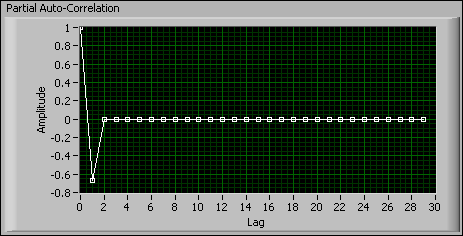The value of the Partial Auto-Correlation plot in the previous figure becomes zero when lag equals two or greater. Therefore, a suitable AR order for this model is two.

Instead of computing the partial auto-correlation function for a time series, you can use a set of model-selection criteria to estimate the model order. From a least-square fitting standpoint, the higher the model order, the better the model fits the time series, because a high-order model has more degrees of freedom. However, an unnecessarily high-order may introduce spurious spectral artifacts in the resulting response. As a result, the criteria you use to assess the model order therefore must not only rely on the model-fitting error but also incorporate a penalty when the order increases. Different selections for the penalty determine different criteria.

### Akaike's Information Criterion

The Akaike's Information Criterion (AIC) is a weighted estimation error based on the unexplained variation of a given time series with a penalty term when exceeding the optimal number of parameters to represent the system. For the AIC, an optimal model is the one that minimizes the following equation: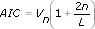where L is the number of data points in a time series, n is the model order, and Vn is the prediction error.

### Bayesian Information Criterion

The Bayesian Information Criterion (BIC) replaces the term 2n in the AIC with the expression (n + nln(L)). The BIC penalizes excess model order more severely than the AIC does. For the BIC, an optimal model is the one that minimizes the following equation: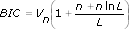### Final Prediction Error Criterion

The Final Prediction Error Criterion (FPE) estimates the model-fitting error when you use the model to predict new outputs. For the FPE, an optimal model is the one that minimizes the following equation: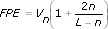### Minimal Description Length Criterion

The Minimal Description Length Criterion (MDL) is based on Vn plus a penalty for the number of terms used. For the MDL, an optimal model is the one that minimizes the following equation: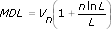### Phi Criterion

The Phi Criterion (PIC) generates an optimal model that minimizes the following equation: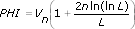Use the TSA AR Modeling Order VI to estimate a suitable AR model order for a time series. This VI implements the partial auto-correlation function and uses the AIC, BIC, FPE, MDL, and PIC methods to search for the optimal model order in the range of interest.

Refer to the AR Order Estimation VI in the labview\examples\Time Series Analysis\TSAGettingStarted directory for an example that demonstrates how to obtain the AR model order of a univariate time series.

If you use the Time Series Modeling Express VI to build an AR model interactively, this Express VI computes the value of the specified criterion function within the order range and highlights the optimal order in the Criterion Function graph, as shown in the following figure. You also can select a different order manually on the Criterion Function graph.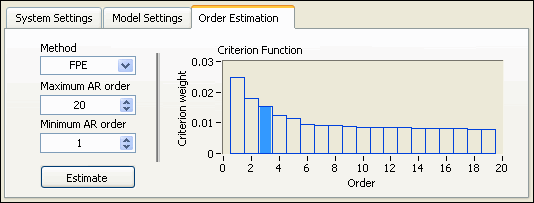Complete the following steps to select an appropriate AR model for AR models you build by using the Time Series Modeling Express VI.

1. In the Configure Time Series Modeling dialog box, click the Model Settings tab and select AR in Type.
2. Click the Order Estimation tab. On the Order Estimation page, complete the following steps to estimate a suitable AR order for the time series:
1. In the Method list, select an appropriate criterion function.
2. Specify an appropriate AR order range in the Maximum AR order box and the Minimum AR order box.
3. Click the Estimate button.
3. Click the Model Settings tab. In the AR order box, specify the value estimated in Step 2. In the Method of AR list, specify an appropriate estimation method.
4. Verify the estimate model by watching the Noise auto-correlation plot of model error. If the estimated model is suitable for the univariate time series, the values of the auto-correlation without lab t being zero fall into the confidence interval.